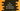# 2 different C# program to check if all numbers in an array are even or odd## C# program to check if all numbers in an array are even or odd in 2 different ways:

In this C# program, we will learn how to check if all numbers in an array are even or odd. So, one number array is given, write one C# program to check if all numbers in that array are even or odd.

We can use a for loop or any other loop to iterate through the array elements one by one and verify if all numbers in that array are even or odd. Another way to do this is by using Array.Exists method.

Let’s take a look at the programs.

### By using a loop:

In this method, we will use a loop that will iterate through the numbers in the array one by one. If it finds any one element which is not odd or even, it returns False.

Below is the complete program:

``````using System;
public class Program {
private static bool allOdd(int[] arr) {
for (int i = 0; i < arr.Length; i++) {
if (arr[i] % 2 == 0) {
return false;
}
}
return true;
}

private static bool allEven(int[] arr) {
for (int i = 0; i < arr.Length; i++) {
if (arr[i] % 2 != 0) {
return false;
}
}
return true;
}

public static void Main()

{
int[] firstArr = new int[] {
1,
2,
3,
4,
5
};
int[] secondArr = new int[] {
1,
3,
5,
7,
9
};
int[] thirdArr = new int[] {
2,
4,
6,
8,
10
};

Console.WriteLine("firstArr all even: " + allEven(firstArr));
Console.WriteLine("secondArr all even: " + allEven(secondArr));
Console.WriteLine("thirdArr all even: " + allEven(thirdArr));
Console.WriteLine("firstArr all odd: " + allOdd(firstArr));
Console.WriteLine("secondArr all odd: " + allOdd(secondArr));
Console.WriteLine("thirdArr all odd: " + allOdd(thirdArr));
}

}``````

Here,

• allOdd and allEven methods are used to check if all numbers in an array are odd and all numbers in an array are even respectively.
• Both of these methods take one array as the input and return one boolean value.
• Both methods are working in the same way. For example, allEven checks if all numbers in the array are even or not. It loops through the elements of the array one by one. If it finds any number which is not even, i.e. number % 2 is not equal to 0, it returns false. After the loop ends, it returns true, i.e. all numbers are even.
• Similar to allEven, allOdd is another method to check if all elements of an are odd or not.
• Inside Main, we have initialized three arrays: firstArr, secondArr and thirdArr. Here, firstArr holds both odd and even numbers. secondArr holds only odd numbers and thirdArr holds only even numbers.

If you run this program, it will print the below output:

``````firstArr all even: False
secondArr all even: False
thirdArr all even: True
firstArr all odd: False
secondArr all odd: True
thirdArr all odd: False``````

### Method 2: By using Array.Exists:

We can use Array.Exists. This method takes one array as the first argument and one predicate as the second argument. The predicate is applied to each element in the array and returns true if any element found which matches the predicate.

We can write the above program as like below:

``````using System;

public class Program
{
private static bool allOdd(int[] arr) {
return !Array.Exists(arr, element => element % 2 == 0);
}

private static bool allEven(int[] arr) {
return !Array.Exists(arr, element => element % 2 != 0);
}

public static void Main() {
int[] firstArr = new int[] {
1,
2,
3,
4,
5
};
int[] secondArr = new int[] {
1,
3,
5,
7,
9
};
int[] thirdArr = new int[] {
2,
4,
6,
8,
10
};

Console.WriteLine("firstArr all even: " + allEven(firstArr));
Console.WriteLine("secondArr all even: " + allEven(secondArr));
Console.WriteLine("thirdArr all even: " + allEven(thirdArr));
Console.WriteLine("firstArr all odd: " + allOdd(firstArr));
Console.WriteLine("secondArr all odd: " + allOdd(secondArr));
Console.WriteLine("thirdArr all odd: " + allOdd(thirdArr));
}
}``````

Here,

• allEven is checking if any element which is not divisible by 2 exists in the array or not. It returns reverse or not(!) value of that.
• Similar to allEven, allOdd is doing the reverse.

It will print the same output.

``````firstArr all even: False
secondArr all even: False
thirdArr all even: True
firstArr all odd: False
secondArr all odd: True
thirdArr all odd: False``````